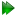The MMQ CR72 model

This is the analytic CR72 model for 2-site exchange on most times scales (Section 11.3.4 on page) extended for multiple types of multiple quantum data (MMQ) by Korzhnev et al. (2004a). It is selected by setting the model to `MMQ CR72'. The simple constraint pA > pB is used to halve the optimisation space, as both sides of the limit are mirror image spaces. The equation for the exchange process is

 R2eff = ℜ(λ1) -ln(Q), (11.44)

where

 λ1 = R2, MQ0 +- νCPMGcosh-1D+cosh(η+) - D-cos(η-), (11.45) D± =±1 +, (11.46) η± = 2±Ψ +, (11.47) Ψ =ıΔωH + pAkex - pBkex- Δω2 +4pApBkex2, (11.48) ζ = - 2ΔωıΔωH + pAkex - pBkex, (11.49)

and where

 Q = ℜ1 - mD+2 + mD+mZ+ - mZ+2 +, (11.50)

and

 mD± = ±z± +2Δω, (11.51) mZ= ±d± -2Δω, (11.52)

and

 d± =ΔωH + Δω±ıkex, (11.53) z± =ΔωH - Δω±ıkex. (11.54)

The symbol δ is half of τCPMG or

 δ =. (11.55)

The references for this model are: